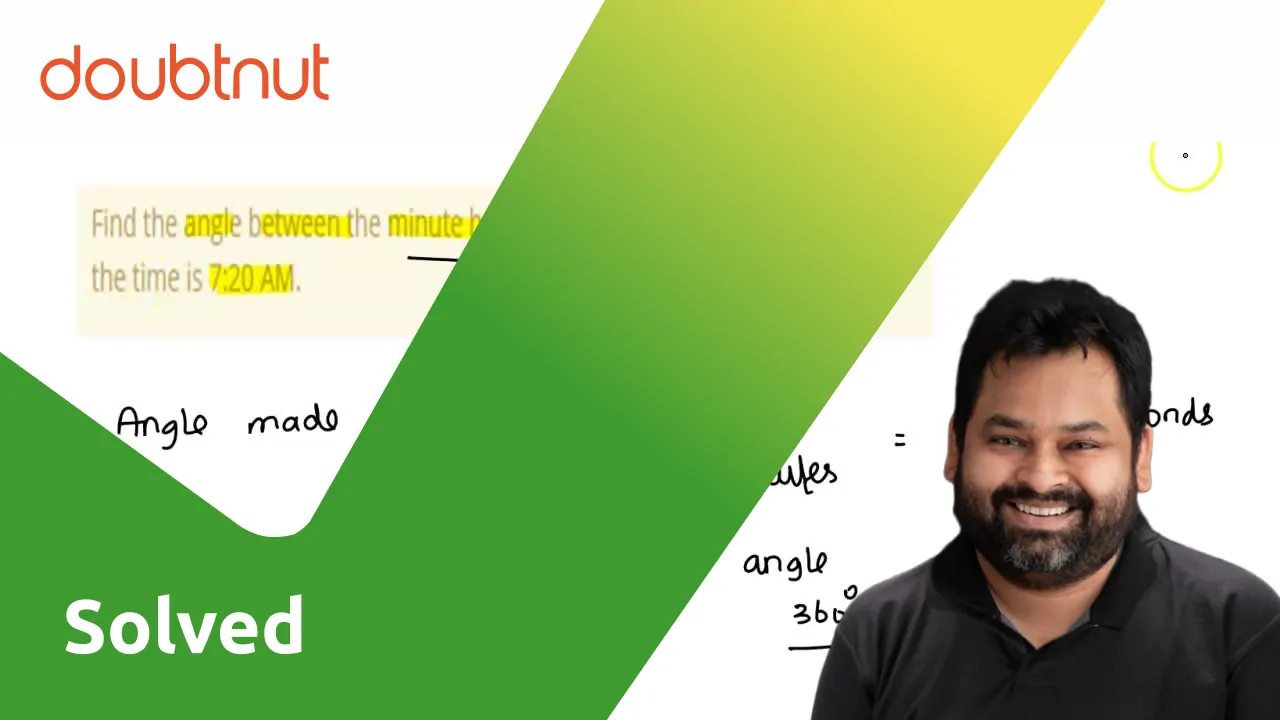Doubtnut App - Get 50 Lakh+ video solutions better than GoogleInstall Now

# Find the angle between the minute hand and the hour hand of a clock when the time is 7:20 AM.Solution in Tamil

Text Solution
Verified by Experts

## Angle made by minute hand in 20 minutes =36060∗20=120∘ Now, hour hand makes an angle 360∘ in 720 minutes. Total number of minutes at 7:20 AM =7∗60+20=440 So, angle made by hour hand at 7:20 AM =360720∗440=220∘ ∴ Angle made between the minute hand and the hour hand at 7:20 AM =220−120=100∘